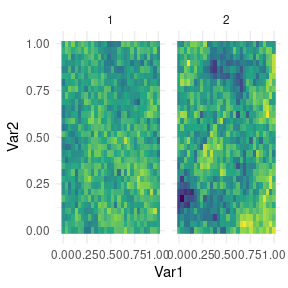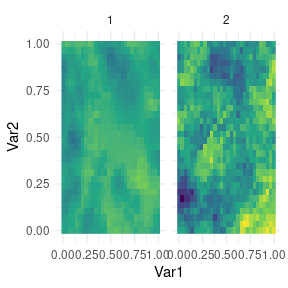# Spatial regression with bivariate misaligned outcomes

The spamtree package is built to run multivariate spatial regressions based on spatial multivariate trees and using a non-separable cross-covariance function on latent dimensions. In this vignette we simulate two spatially referenced outcomes.

library(abind)
library(magrittr)
library(dplyr)
#>
#> Attaching package: 'dplyr'
#> The following objects are masked from 'package:stats':
#>
#>     filter, lag
#> The following objects are masked from 'package:base':
#>
#>     intersect, setdiff, setequal, union
library(ggplot2)
library(spamtree)

set.seed(2021)

SS <- 30 # coordinate values for jth dimension
n <- SS^2 # total n. locations, including missing ones

xlocs <- seq(0.0, 1, length.out=SS)
coords <- expand.grid(xlocs, xlocs) %>%
as.data.frame() %>%
arrange(Var1, Var2)

c1 <- coords %>% mutate(mv_id=1)
c2 <- coords %>% mutate(mv_id=2)

coords <- bind_rows(c1, c2)

coords_q <- coords %>% dplyr::select(-mv_id)
cx <- coords_q %>% as.matrix()
ix <- 1:nrow(cx) - 1
mv_id <- coordsmv_id q <- 2 sigma.sq <- 1 tau.sq <- c(.03, .1) tausq_long <- rep(0, nrow(cx)) tausq_long[mv_id == 1] <- tau.sq tausq_long[mv_id == 2] <- tau.sq # some true values for the non-separable multivariate cross-covariance implemented here ai1 <- c(1, 1.5) ai2 <- c(.1, .51) phi_i <- c(1, 2) thetamv <- 5 Dmat <- matrix(0, q, q) Dmat[2,1] <- 1 Dmat[upper.tri(Dmat)] <- Dmat[lower.tri(Dmat)] X <- cbind(rnorm(nrow(coords)), rnorm(nrow(coords))) B <- c(-.9, .05) # generate covariance matrix for full GP system.time({ CC <- CrossCovarianceAG10(cx, mv_id, cx, mv_id, ai1, ai2, phi_i, thetamv, Dmat) }) #> user system elapsed #> 0.065 0.015 0.081 LC <- t(chol(CC)) # sample the outcomes at all locations y_full <- X %*% B + LC %*% rnorm(nrow(cx)) + sqrt(tausq_long) * rnorm(nrow(cx)) rm(list=c("CC", "LC")) # make some na: 0=na # (this also creates some misalignment) lna <- rep(1, nrow(coords)) lna[((coords_qVar1 > .4) & (coords_q$Var1 < .9) & (coords_q$Var2 < .7) & (coords_q$Var2 > .4)) & (mv_id == 1)] <- NA lna[((coords_q$Var1 > .2) & (coords_q$Var1 < .7) & (coords_q$Var2 < .7) & (coords_q$Var2 > .4)) & (mv_id == 2)] <- NA y <- y_full * lna simdata <- coords %>% cbind(y) %>% as.data.frame() We now run spamtree. In practice the data size would be much larger, and we would run many more MCMC iterations.  # prepare for spamtrees mcmc_keep <- 200 mcmc_burn <- 200 mcmc_thin <- 2 spamtree_done <- spamtree(y, X, cx, mv_id, mcmc = list(keep=mcmc_keep, burn=mcmc_burn, thin=mcmc_thin), num_threads = 10, verbose=TRUE) #> Building reference set. #> Branching the tree 1 ( 1 ) 2 ( 2 ) 3 ( 3 ) 4 ( 4 ). #> Finalizing with leaves. #> Building graph. #> Running MCMC for 600 iterations. #> 10.0% 226ms (total: 257ms) ~ MCMC acceptance 9.50% (total: 31.15%) #> 20.0% 199ms (total: 456ms) ~ MCMC acceptance 12.50% (total: 20.66%) #> 30.0% 193ms (total: 649ms) ~ MCMC acceptance 14.00% (total: 15.47%) #> 40.0% 200ms (total: 850ms) ~ MCMC acceptance 7.00% (total: 11.62%) #> 50.0% 196ms (total: 1047ms) ~ MCMC acceptance 5.00% (total: 11.30%) #> 60.0% 201ms (total: 1249ms) ~ MCMC acceptance 6.50% (total: 11.08%) #> 70.0% 203ms (total: 1452ms) ~ MCMC acceptance 8.50% (total: 10.69%) #> 80.0% 209ms (total: 1661ms) ~ MCMC acceptance 11.00% (total: 10.81%) #> 90.0% 216ms (total: 1878ms) ~ MCMC acceptance 15.00% (total: 12.57%) #> MCMC done [2074ms] And finally we do some postprocessing and plot the predictions for both outcomes, and the latent process. # predictions y_out <- spamtree_done$yhat_mcmc %>%
abind(along=3) %>% [(,1,) %>%
apply(1, mean)

w_out <- spamtree_done$w_mcmc %>% abind(along=3) %>% [(,1,) %>% apply(1, mean) outdf <- spamtree_done$coordsinfo %>%
rename(mv_id = sort_mv_id) %>%
cbind(data.frame(w_spamtree = w_out,
y_spamtree = y_out))

# plot predictions
outdf %>%
ggplot(aes(Var1, Var2, fill=y_spamtree)) +
geom_raster() +
facet_grid(~mv_id) +
scale_fill_viridis_c() +
theme_minimal() + theme(legend.position="none")# plot latent process
outdf %>%
ggplot(aes(Var1, Var2, fill=w_spamtree)) +
geom_raster() +
facet_grid(~mv_id) +
scale_fill_viridis_c() +
theme_minimal() + theme(legend.position="none")